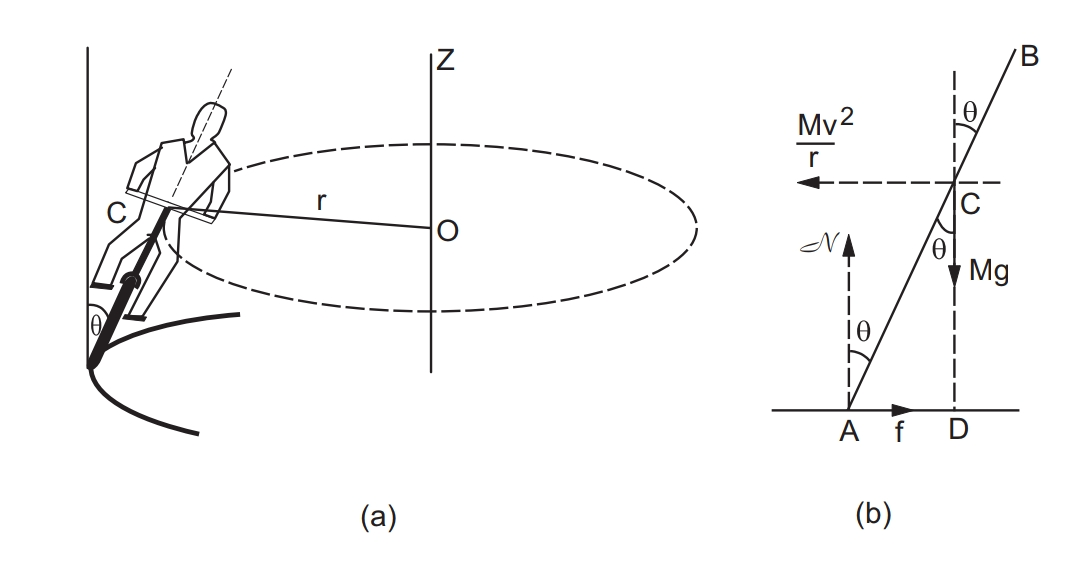# Bending of a cyclist on a horizontal turn reason

When a cyclist travels along a circular path, its velocity changes continuously. Naturally, an external force always acts on the cycle towards the center of the path. The external working force on the cycle to maintain the circular motion is centripetal force and due to this centripetal force, the cyclist faces a bend on a horizontal turn.

# Bending of a cyclist on a horizontal turn derivation and explanation

Suppose a cyclist is going at a speed v on a circular horizontal road of radius r which is not banked. Consider the cycle and the rider together as the system. The center of mass C of the system is going in a circle with the center at O and radius r as shown in the given figure.Let us choose O as the origin, OC as the X-axis, and vertically upward as the Z-axis. This frame is rotating at an angular speed ω = v / r about the Z-axis. In this frame, the system is at rest. Since we are working from a rotating frame of reference, we will have to apply a centripetal force on each particle. The net centripetal force on the system will be Mω2r = Mv2 / r, where M is the total mass of the system. This force will act through the center of mass. Since the system is at rest in this frame, no other pseudo force is needed.

given Figure shows the forces. The cycle is bent at an angle with the vertical.

The forces are

• ( i ) weight Mg,
• ( ii ) normal force N,
• ( iii ) friction f and,
• ( iv ) centripetal force Mv2 / r.

In the frame considered, the system is at rest. Thus, the total external force and the total external torque must be zero.

Let us consider the torques of all the forces about point A. The torques of N and f about A are zero because these forces pass through A. The torque of Mg about A is Mg ( AD ) in the clockwise direction and that of Mv2/r is mv2/r( CD ) in the anti-clockwise direction.

For rotational equilibrium,

Mg ( AD ) = mv2/r ( CD )